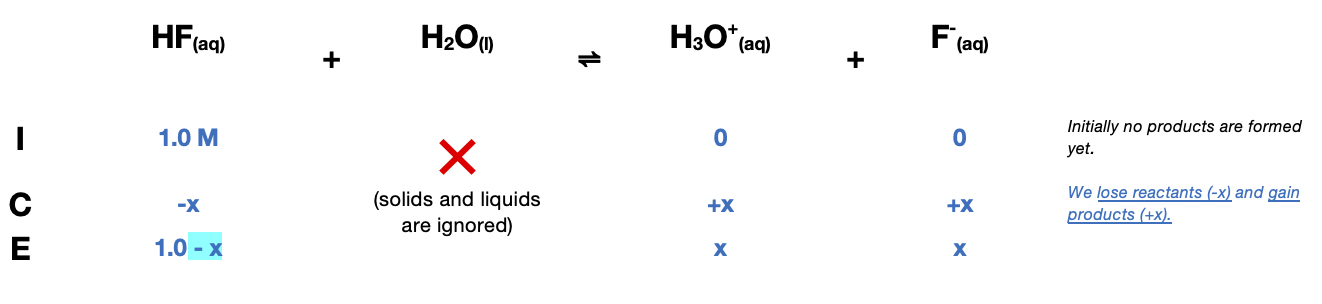# Problem: Calculate the pH of a solution that contains 1.0 M HF and 1.0 M HOC 6H5. Also calculate the concentration of OC6H5- in this solution at equilibrium.

###### FREE Expert Solution$\overline{){{\mathbf{K}}}_{{\mathbf{a}}}{\mathbf{=}}\frac{\mathbf{products}}{\mathbf{reactants}}{\mathbf{=}}\frac{\left[{H}_{3}{O}^{+}\right]\left[{F}^{-}\right]}{\left[\mathrm{HF}\right]}}$

${\mathbf{6}}{\mathbf{.}}{\mathbf{76}}{\mathbf{×}}{{\mathbf{10}}}^{\mathbf{-}\mathbf{4}}{\mathbf{=}}\frac{\left(x\right)\left(x\right)}{1-x}\phantom{\rule{0ex}{0ex}}\phantom{\rule{0ex}{0ex}}{\mathbf{6}}{\mathbf{.}}{\mathbf{76}}{\mathbf{×}}{{\mathbf{10}}}^{\mathbf{-}\mathbf{4}}{\mathbf{=}}\frac{{x}^{2}}{1-x}$

Since Ka <<1, we can ignore x in the denominator.

${\mathbf{6}}{\mathbf{.}}{\mathbf{76}}{\mathbf{×}}{{\mathbf{10}}}^{\mathbf{-}\mathbf{4}}{\mathbf{=}}\frac{{x}^{2}}{1-\overline{)x}}\phantom{\rule{0ex}{0ex}}\phantom{\rule{0ex}{0ex}}{\mathbf{6}}{\mathbf{.}}{\mathbf{76}}{\mathbf{×}}{{\mathbf{10}}}^{\mathbf{-}\mathbf{4}}{\mathbf{=}}\frac{{\mathbf{x}}^{\mathbf{2}}}{\mathbf{1}}\phantom{\rule{0ex}{0ex}}\phantom{\rule{0ex}{0ex}}\sqrt{{\mathbf{x}}^{\mathbf{2}}}{\mathbf{=}}\sqrt{\mathbf{6}\mathbf{.}\mathbf{76}\mathbf{×}{\mathbf{10}}^{\mathbf{-}\mathbf{4}}}$

89% (45 ratings)###### Problem Details

Calculate the pH of a solution that contains 1.0 M HF and 1.0 M HOC 6H5. Also calculate the concentration of OC6H5- in this solution at equilibrium.

Frequently Asked Questions

What scientific concept do you need to know in order to solve this problem?

Our tutors have indicated that to solve this problem you will need to apply the Weak Acids concept. If you need more Weak Acids practice, you can also practice Weak Acids practice problems.

What professor is this problem relevant for?

Based on our data, we think this problem is relevant for Professor Hashim's class at UCF.

What textbook is this problem found in?

Our data indicates that this problem or a close variation was asked in Chemistry: An Atoms First Approach - Zumdahl Atoms 1st 2nd Edition. You can also practice Chemistry: An Atoms First Approach - Zumdahl Atoms 1st 2nd Edition practice problems.